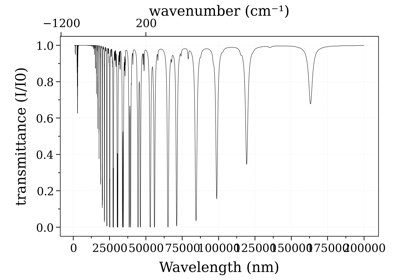# Line-of-sight module¶

This module takes several `Spectrum` objects as an input and combines then along the line-of-sight (`SerialSlabs()`) or at the same spatial position (`MergeSlabs()`), to reproduce line-of-sight experiments

## How to combine slabs?¶

### Along the line-of-sight¶

Use the `SerialSlabs()` function:

```s1 = calc_spectrum(...)
s2 = calc_spectrum(...)
s3 = SerialSlabs(s1, s2)
```

You can also use the `>` operator. The previous line is equivalent to:

```s3 = s1 > s2
```

### At the same spatial position¶

Use the `MergeSlabs()` function:

Merge two spectra calculated with different species (true only if broadening coefficient dont change much):

```from radis import calc_spectrum, MergeSlabs
s1 = calc_spectrum(...)
s2 = calc_spectrum(...)
s3 = MergeSlabs(s1, s2)
```

You can also use the `//` operator. The previous line is equivalent to:

```s3 = s1 // s2
```Calculate a large spectrum by part

Calculate a large spectrum by part

## Practical Examples¶

Below are some practical examples of the use of the Line-of-sight module:

### Build a large spectrum¶

If you want to calculate a spectrum on a very large spectral range which cannot be handled in memory at once, you can calculate partial, non-overlapping spectral ranges and use `MergeSlabs()` to combine them. In that case, we tell `MergeSlabs()` to use the full spectral range and that the partial spectra are transparent outside of their definition range:

```from radis import load_spec, MergeSlabs
spectra = []
for f in ['spec1.spec', 'spec2.spec', ...]:  # precomputed spectra
s = MergeSlabs(*spectra, resample='full', out='transparent')
s.plot()
```

### Get the contribution of each slab along the LOS¶

Let’s say you have a total line of sight:

```s_los = s1 > s2 > s3
```

If you want to get the contribution of `s2` to the line-of-sight emission, you need to discard the emission of `s3` but take into account its absorption. This is done using the `PerfectAbsorber()` function, which returns a new Spectrum with all the emission features set to 0:

```from radis import PerfectAbsorber
And the contribution of `s1` would be:
```(s1 > PerfectAbsorber(s2>s3)).plot('radiance_noslit')